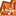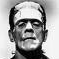# QlikView App Dev

Discussion Board for collaboration related to QlikView App Development.

Announcements
Become an analytics expert with Qlik's new 15 week course: Applied Data Analytics using Qlik Sense. READ MORE
cancel
Showing results for
Did you mean:Contributor III

## Problem export excel number format num(..)

Hi everyone,

I'm sorry if my issue was already solved in another post but I couldn't find the answer to my problem.

Actually here it is:

I have a table where I'm calculating different stuff as Margins, Volumes of sales and so on.

For one of the indicator I'm calculating volumes for diffents things (volume in terms of turnover or volume in term of number of sales for instance).

As I cannot use one single format for the numbers I use the

- NUM(expression, format-code ('# ##0')) for numerical

- NUM(expression, format-code ('# ##0 €')) for monetary units

Everything's fine so far but the problem is when I export the table on Excel.

Excel doesn't recognize the format I've defined and display the values as Text which is bad when we want to use the Excel file for other calculation.

Do you have any idea of how I can deal with that.

20 RepliesNot applicable

I am having the same issue and have searched the forum for an answer.  As you stated, because the expression must be in multiple formats depending on the filter choices, you cannot solve the number export issue by changing the Number Format Settings in the document properties.  I had no issues in v9 and have just recently upgraded to v11.  I have contacted QV Support and have not received an answer yet.  If you received an answer outside of the forum, I would greatly appreciate a reply with the answer.  Thanks so much!

Document Expression:

IF(pipecharttype='\$',num(sum(if(\$(vTimeDimension)=\$(vTimeDimension3),estimatedvalue))-sum(if (\$(vTimeDimension3)=\$(vTimeDimension2),estimatedvalue)),'\$##,##0;(\$##,##0)'),num(count(if(\$(vTimeDimension)=\$(vTimeDimension3),opportunityid))-count(if (\$(vTimeDimension3)=\$(vTimeDimension2),opportunityid)),'#,###0;(#,###0)'))Not applicable

I am having the same issue and have searched the forum for an answer.  As you stated, because the expression must be in multiple formats depending on the filter choices, you cannot solve the number export issue by changing the Number Format Settings in the document properties.  I had no issues in v9 and have just recently upgraded to v11.  I have contacted QV Support and have not received an answer yet.  If you received an answer outside of the forum, I would greatly appreciate a reply with the answer.  Thanks so much!

Document Expression:

IF(pipecharttype='\$',num(sum(if(\$(vTimeDimension)=\$(vTimeDimension3),estimatedvalue))-sum(if (\$(vTimeDimension3)=\$(vTimeDimension2),estimatedvalue)),'\$##,##0;(\$##,##0)'),num(count(if(\$(vTimeDimension)=\$(vTimeDimension3),opportunityid))-count(if (\$(vTimeDimension3)=\$(vTimeDimension2),opportunityid)),'#,###0;(#,###0)'))Not applicable

I am having the same issue and have searched the forum for an answer.  As you stated, because the expression must be in multiple formats depending on the filter choices, you cannot solve the number export issue by changing the Number Format Settings in the document properties.  I had no issues in v9 and have just recently upgraded to v11.  I have contacted QV Support and have not received an answer yet.  If you received an answer outside of the forum, I would greatly appreciate a reply with the answer.  Thanks so much!

Document Expression:

IF(pipecharttype='\$',num(sum(if(\$(vTimeDimension)=\$(vTimeDimension3),estimatedvalue))-sum(if (\$(vTimeDimension3)=\$(vTimeDimension2),estimatedvalue)),'\$##,##0;(\$##,##0)'),num(count(if(\$(vTimeDimension)=\$(vTimeDimension3),opportunityid))-count(if (\$(vTimeDimension3)=\$(vTimeDimension2),opportunityid)),'#,###0;(#,###0)'))Not applicable

I am having the same issue and have searched the forum for an answer.  As you stated, because the expression must be in multiple formats depending on the filter choices, you cannot solve the number export issue by changing the Number Format Settings in the document properties.  I had no issues in v9 and have just recently upgraded to v11.  I have contacted QV Support and have not received an answer yet.  If you received an answer outside of the forum, I would greatly appreciate a reply with the answer.  Thanks so much!

Document Expression:

IF(pipecharttype='\$',num(sum(if(\$(vTimeDimension)=\$(vTimeDimension3),estimatedvalue))-sum(if (\$(vTimeDimension3)=\$(vTimeDimension2),estimatedvalue)),'\$##,##0;(\$##,##0)'),num(count(if(\$(vTimeDimension)=\$(vTimeDimension3),opportunityid))-count(if (\$(vTimeDimension3)=\$(vTimeDimension2),opportunityid)),'#,###0;(#,###0)'))Master II

After exporting to Excel, you can convert the text to values in Excel via a macro.Contributor III
Author

I agree with the idea of a macro in Excel but in my case I just chose to use the standard Number Format Settings in the document properties so that every kind of KPI has a numeric format.

I just added in the KPI label a dynamic label giving the correct format depending on the selection.

So when you export in Excel everything's fine and the user can still have a clue of the measuring unit.

However Melanie, if you have any answer from QV support please let me know.Specialist II

Hi:

It was a QV bug, in the next version it will be solved ... read this pdfContributor III
Author

Thanks JoaquinIr I'll have a look at this doc and I'll think about upgrading in SR2...Specialist II

OK ... but SR2 is closed, not released for the public.